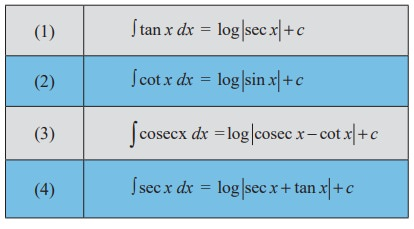Home | | Maths 11th std | Important Results of Integral Calculus

# Important Results of Integral Calculus

Mathematics : Integral Calculus - Important Results of Integral Calculus

Important ResultsExample 11.31

Integrate the following with respect to x.

(i) Ōł½ tan x dx

(ii) Ōł½ cot x dx

(iii) Ōł½ cosec x dx

(iv) Ōł½ sec x dx

SolutionThus the following are the important standard results.Example 11.32

Integrate the following with respect to x.EXERCISE 11.6

Integrate the following with respect to xStudy Material, Lecturing Notes, Assignment, Reference, Wiki description explanation, brief detail
11th Mathematics : UNIT 11 : Integral Calculus : Important Results of Integral Calculus |